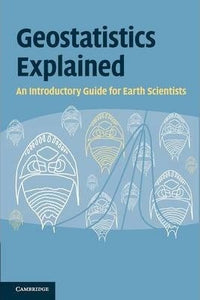# Geostatistics Explained : An Introductory Guide for Earth Scientists by Mckillup, Steve

• R 400.00
Unit price per
Tax included.

ISBN: 9780521746564

Cambridge University Press | 28 July 2014

Paperback | 414 pages

A reader-friendly introduction to geostatistics for students and researchers struggling with statistics. Using simple, clear explanations for introductory and advanced material, it demystifies complex concepts and makes formulas and statistical tests easy to apply. Beginning with a critical evaluation of experimental and sampling design, the book moves on to explain essential concepts of probability, statistical significance and type 1 and type 2 error. An accessible graphical explanation of analysis of variance (ANOVA) leads onto advanced ANOVA designs, correlation and regression, and non-parametric tests including chi-square. Finally, it introduces the essentials of multivariate techniques, multi-dimensional scaling and cluster analysis, analysis of sequences and concepts of spatial analysis. Illustrated with wide-ranging examples from topics across the Earth and environmental sciences, Geostatistics Explained can be used for undergraduate courses or for self-study and reference. Worked examples at the end of each chapter reinforce a clear understanding of the statistical tests and their applications.